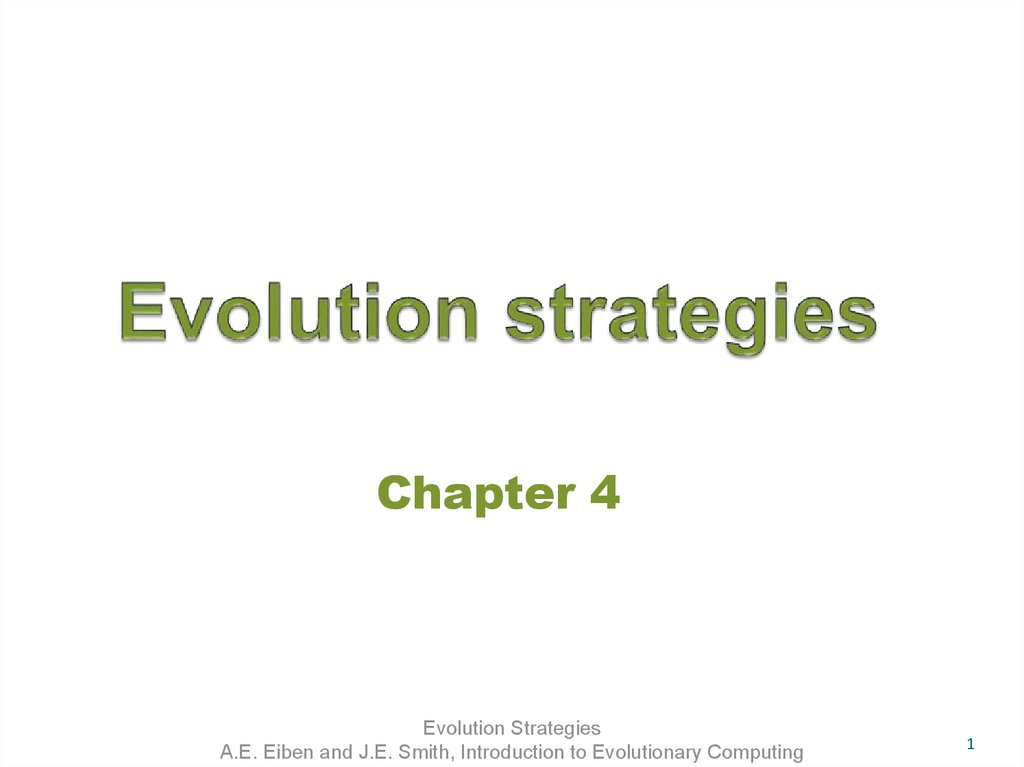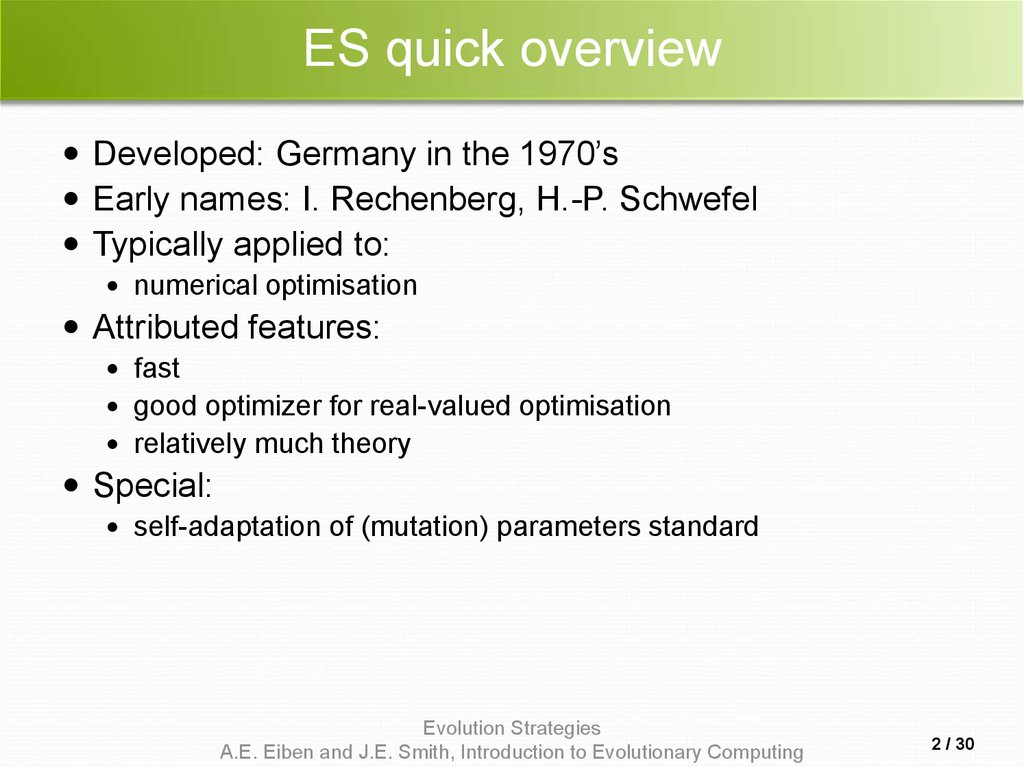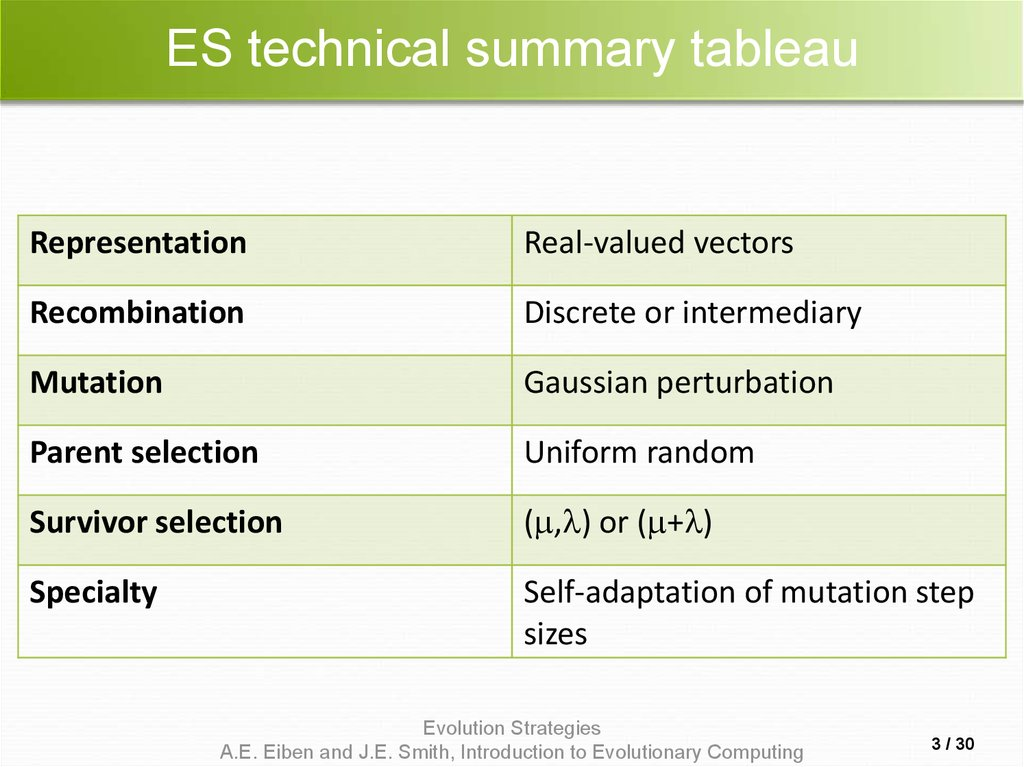# Evolution strategies

## 1. Evolution strategies

Chapter 4
Evolution Strategies
A.E. Eiben and J.E. Smith, Introduction to Evolutionary Computing
1

## 2. ES quick overview

Developed: Germany in the 1970’s
Early names: I. Rechenberg, H.-P. Schwefel
Typically applied to:
numerical optimisation
Attributed features:
fast
good optimizer for real-valued optimisation
relatively much theory
Special:
Evolution Strategies
A.E. Eiben and J.E. Smith, Introduction to Evolutionary Computing
2 / 30

## 3. ES technical summary tableau

Representation
Real-valued vectors
Recombination
Discrete or intermediary
Mutation
Gaussian perturbation
Parent selection
Uniform random
Survivor selection
( , ) or ( + )
Specialty
sizes
Evolution Strategies
A.E. Eiben and J.E. Smith, Introduction to Evolutionary Computing
3 / 30

## 4. Introductory example

n
Task: minimimise f : R R
Algorithm: “two-membered ES” using
n
Vectors from R directly as chromosomes
Population size 1
Only mutation creating one child
Greedy selection
Evolution Strategies
A.E. Eiben and J.E. Smith, Introduction to Evolutionary Computing
4 / 30

## 5. Introductory example: pseudocde

Set t = 0
Create initial point xt = x1t,…,xnt
REPEAT UNTIL (TERMIN.COND satisfied) DO
Draw zi from a normal distr. for all i = 1,…,n
yit = xit + zi
IF f(xt) < f(yt) THEN xt+1 = xt
ELSE xt+1 = yt
FI
Set t = t+1
OD
Evolution Strategies
A.E. Eiben and J.E. Smith, Introduction to Evolutionary Computing
5 / 30

## 6. Introductory example: mutation mechanism

z values drawn from normal distribution N( , )
mean is set to 0
variation is called mutation step size
is varied on the fly by the “1/5 success rule”:
This rule resets after every k iterations by
= /c
= •c
=
if ps > 1/5
if ps < 1/5
if ps = 1/5
where ps is the % of successful mutations, 0.8 c 1
Evolution Strategies
A.E. Eiben and J.E. Smith, Introduction to Evolutionary Computing
6 / 30

## 7. Illustration of normal distribution

Evolution Strategies
A.E. Eiben and J.E. Smith, Introduction to Evolutionary Computing
7 / 30

## 8. Another historical example: the jet nozzle experiment

Evolution Strategies
A.E. Eiben and J.E. Smith, Introduction to Evolutionary Computing
8 / 30

## 9. The famous jet nozzle experiment (movie)

Evolution Strategies
A.E. Eiben and J.E. Smith, Introduction to Evolutionary Computing
9 / 30

## 10.

Representation
Chromosomes consist of three parts:
Object variables: x1,…,xn
Strategy parameters:
Mutation step sizes: 1,…, n
Rotation angles: 1,…, n
Not every component is always present
Full size: x1,…,xn, 1,…, n , 1,…, k
where k = n(n-1)/2 (no. of i,j pairs)
Evolution Strategies
A.E. Eiben and J.E. Smith, Introduction to Evolutionary Computing
10 / 30

## 11. Mutation

Main mechanism: changing value by adding
random noise drawn from normal distribution
x’i = xi + N(0, )
Key idea:
is part of the chromosome x1,…,xn,
is also mutated into ’ (see later how)
Thus: mutation step size is coevolving with the
solution x
Evolution Strategies
A.E. Eiben and J.E. Smith, Introduction to Evolutionary Computing
11 / 30

## 12. Mutate  first

Mutate first
Net mutation effect: x, x’, ’
Order is important:
first ’ (see later how)
then x x’ = x + N(0, ’)
Rationale: new x’ , ’ is evaluated twice
Primary: x’ is good if f(x’) is good
Secondary: ’ is good if the x’ it created is good
Step-size only survives through “hitch-hiking”
Reversing mutation order this would not work
Evolution Strategies
A.E. Eiben and J.E. Smith, Introduction to Evolutionary Computing
12 / 30

## 13. Mutation case 1: Uncorrelated mutation with one 

Mutation case 1:
Uncorrelated mutation with one
Chromosomes: x1,…,xn,
’ = • exp( • N(0,1))
x’i = xi + ’ • N(0,1)
Typically the “learning rate” 1/ n½
And we have a boundary rule ’ < 0 ’ = 0
Evolution Strategies
A.E. Eiben and J.E. Smith, Introduction to Evolutionary Computing
13 / 30

## 14. Mutants with equal likelihood

Circle: mutants having the same chance to be created
Evolution Strategies
A.E. Eiben and J.E. Smith, Introduction to Evolutionary Computing
14 / 30

## 15. Mutation case 2: Uncorrelated mutation with n ’s

Mutation case 2:
Uncorrelated mutation with n ’s
Chromosomes: x1,…,xn, 1,…, n
’i = i • exp( ’ • N(0,1) + • Ni (0,1))
x’i = xi + ’i • Ni (0,1)
Two learning rate parameters:
’ overall learning rate
coordinate wise learning rate
1/(2 n)½ and 1/(2 n½) ½
Boundary rule: i’ < 0 i’ = 0
Evolution Strategies
A.E. Eiben and J.E. Smith, Introduction to Evolutionary Computing
15 / 30

## 16. Mutants with equal likelihood

Ellipse: mutants having the same chance to be created
Evolution Strategies
A.E. Eiben and J.E. Smith, Introduction to Evolutionary Computing
16 / 30

## 17. Mutation case 3: Correlated mutations

Chromosomes: x1,…,xn, 1,…, n , 1,…, k
where k = n • (n-1)/2
Covariance matrix C is defined as:
cii = i2
cij = 0 if i and j are not correlated
cij = ½ • ( i2 - j2 ) • tan(2 ij) if i and j are correlated
Note the numbering / indices of the ‘s
Evolution Strategies
A.E. Eiben and J.E. Smith, Introduction to Evolutionary Computing
17 / 30

## 18. Correlated mutations cont’d

The mutation mechanism is then:
’i = i • exp( ’ • N(0,1) + • Ni (0,1))
’j = j + • N (0,1)
x ’ = x + N(0,C’)
x stands for the vector x1,…,xn
C’ is the covariance matrix C after mutation of the values
1/(2 n)½ and 1/(2 n½) ½ and 5°
i’ < 0 i’ = 0 and
| ’j | > ’j = ’j - 2 sign( ’j)
NB Covariance Matrix Adaptation Evolution Strategy
(CMA-ES) is probably the best EA for numerical
optimisation, cf. CEC-2005 competition
Evolution Strategies
A.E. Eiben and J.E. Smith, Introduction to Evolutionary Computing
18 / 30

## 19. Mutants with equal likelihood

Ellipse: mutants having
the same chance to be created
Evolution Strategies
A.E. Eiben and J.E. Smith, Introduction to Evolutionary Computing
19 / 30

## 20. Recombination

Creates one child
Acts per variable / position by either
Averaging parental values, or
Selecting one of the parental values
From two or more parents by either:
Using two selected parents to make a child
Selecting two parents for each position anew
Evolution Strategies
A.E. Eiben and J.E. Smith, Introduction to Evolutionary Computing
20 / 30

## 21. Names of recombinations

Two fixed parents
Two parents selected
for each i
zi = (xi + yi)/2
Local intermediary
Global intermediary
zi is xi or yi chosen
randomly
Local discrete
Global discrete
Evolution Strategies
A.E. Eiben and J.E. Smith, Introduction to Evolutionary Computing
21 / 30

## 22. Parent selection

Parents are selected by uniform random
distribution whenever an operator needs
one/some
Thus: ES parent selection is unbiased - every
individual has the same probability to be selected
Note that in ES “parent” means a population
member (in GA’s: a population member selected
to undergo variation)
Evolution Strategies
A.E. Eiben and J.E. Smith, Introduction to Evolutionary Computing
22 / 30

## 23. Survivor selection

Applied after creating children from the
parents by mutation and recombination
Deterministically chops off the “bad stuff”
Two major variants, distinguished by the basis of
selection:
( , )-selection based on the set of children only
( + )-selection based on the set of parents and
children:
Evolution Strategies
A.E. Eiben and J.E. Smith, Introduction to Evolutionary Computing
23 / 30

## 24. Survivor selection cont’d

( + )-selection is an elitist strategy
( , )-selection can “forget”
Often ( , )-selection is preferred for:
Better in leaving local optima
Better in following moving optima
Using the + strategy bad values can survive in x, too long if
their host x is very fit
Selective pressure in ES is high compared with GAs,
7 • is a traditionally good setting (decreasing over the
last couple of years, 3 • seems more popular lately)
Evolution Strategies
A.E. Eiben and J.E. Smith, Introduction to Evolutionary Computing
24 / 30

Given a dynamically changing fitness landscape
(optimum location shifted every 200 generations)
adjust the mutation step size after every shift !
Evolution Strategies
A.E. Eiben and J.E. Smith, Introduction to Evolutionary Computing
25 / 30

Evolution Strategies
A.E. Eiben and J.E. Smith, Introduction to Evolutionary Computing
26 / 30

> 1 to carry different strategies
> to generate offspring surplus
Not “too” strong selection, e.g., 7 •
( , )-selection to get rid of misadapted ‘s
Mixing strategy parameters by (intermediary)
recombination on them
Evolution Strategies
A.E. Eiben and J.E. Smith, Introduction to Evolutionary Computing
27 / 30

## 28. Example application: the cherry brandy experiment

Task: to create a colour mix yielding a target colour (that of
a well known cherry brandy)
Ingredients: water + red, yellow, blue dye
Representation: w, r, y ,b no self-adaptation!
Values scaled to give a predefined total volume (30 ml)
Mutation: lo / med / hi values used with equal chance
Selection: (1,8) strategy
Evolution Strategies
A.E. Eiben and J.E. Smith, Introduction to Evolutionary Computing
28 / 30

## 29. Example application: cherry brandy experiment cont’d

Fitness: students effectively making the mix and
comparing it with target colour
Termination criterion: student satisfied with mixed
colour
Solution is found mostly within 20 generations
Accuracy is very good
Evolution Strategies
A.E. Eiben and J.E. Smith, Introduction to Evolutionary Computing
29 / 30

## 30. Example application: the Ackley function (Bäck et al ’93)

The Ackley function (here used with n =30):
1 n 2
f ( x) 20 exp 0.2
xi
n i 1
1 n
exp cos( 2 xi ) 20 e
n i 1
Evolution strategy:
Representation:
-30 < xi < 30 (coincidence of 30’s!)
30 step sizes
(30,200) selection
Termination : after 200000 fitness evaluations
Results: average best solution is 7.48 • 10
–8
(very good)
Evolution Strategies
A.E. Eiben and J.E. Smith, Introduction to Evolutionary Computing
30 / 30